# The centric force P is applied to a short post as shown. Knowing that the stresses on plane a-a are σ = −15 ksi and σ = 5 ksi, determine (a) the angle β that plane a-a forms with the horizontal, (b) the maximum compressive stress in the post.

Question-AnswerCategory: Strength of MaterialsThe centric force P is applied to a short post as shown. Knowing that the stresses on plane a-a are σ = −15 ksi and σ = 5 ksi, determine (a) the angle β that plane a-a forms with the horizontal, (b) the maximum compressive stress in the post.

The centric force P is applied to a short post as shown. Knowing that the stresses on plane aa are σ = −15 ksi and σ = 5 ksi, determine (a) the angle β that plane a-a forms with the horizontal, (b) the maximum compressive stress in the post.Step No: 1

Draw the free body diagram of the plane a-a.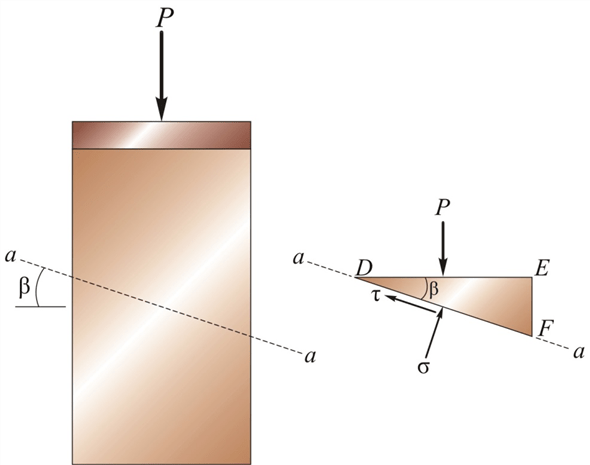Step No: 2

Consider the area of the section of the post on the plane DE be A.
Calculate the area of the cross section of the post on the plane DF by using the equation:Here,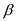is the angle that the plane a-a forms with the horizontal in the counter clockwise direction,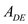is the area of the section of the post on the plane DE and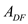is the area of the section of the post on the plane DF.
Substitutefor.Step No: 3

(a)
Calculate the normal force acting on the plane DF by using the equation:Here,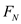is the normal force acting on the plane DF and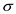is the normal stress on the plane.
Substitutefor.Calculate the shear force acting on the plane DF by using the equation: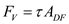Here,is the shear force acting on the plane DF and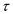is the shear stress on the plane.
Substitute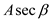for.Step No: 4

Apply force equilibrium equation along the x-axis.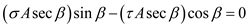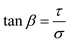Substituteforandfor.Therefore, the anglethat the plane a-a forms with the horizontal in the counter clockwise direction is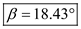.

Step No: 5

Consider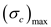is the maximum compressive stress in the post.
Apply force equilibrium equation along the x-axis.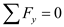Substitute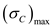for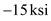forforandfor.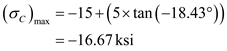Therefore, the maximum compressive stress on the post is